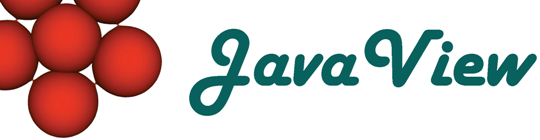Overview  Animation  Classic Surfaces  Parm Surfaces  Curves on Surfaces  Discrete Geodesics  ODE  Platonic Solids  Cycloid  Root Finder  Harmonic Maps  Rivara Bisection  Scalar Field  Weierstrass  Closed Polygon  Elastic Curve  Billiard in an Ellipse  LIC Visualization  Discrete VF  Hodge Splitting  Textured Surface  Surfaces of Rotation  Mean Curvature Flow  Pythagoraen Tree  Julia Sets

## Curves on Hyper Surfaces

Classic curve on parameterized surfaces. The curves are defined via ordinary differential equations on the surfaces. These equations are numerically integrated in JavaView. Select one of various parameterized hyper surfaces and choose among four types of curves on these surfaces: geodesics, shadow lines, asymptotic lines, and principal curvature lines.

The curve are described by differential equations that are integrated with a fourth-order Runge-Kutta method with constant step size.

#### Usage:

Press key `i` and pick a point on the surface. The selected curve is integrated with a step size of length/(discr-1) to a maximum of the given length, or until the edge of the surface is reached.

Controls in the curve panel:
 Choice Choose curve type. Slider "Length" Analytic length of curve. Slider "Polygon Discr" Number of curve vertices. Slider "Direction" Starting Direction of curve. The direction is given by an angle in the tangent space at the selected point. Slider "Thickness" Radius of tube around curve. Slider "Tube Discr" Number of edges of the tube around the curve. Checkbox "Show Tube" Switch tube around the curve to be visible or invisible in display. Checkbox "Show Polygon" Switch the curve polygon to be visible or invisible in display. Checkbox "Show Surface" Switch the surface underlying the curve to be visible or invisible in display. Button "Animate Direction" Get Animation panel to start animation of the initial curve direction.